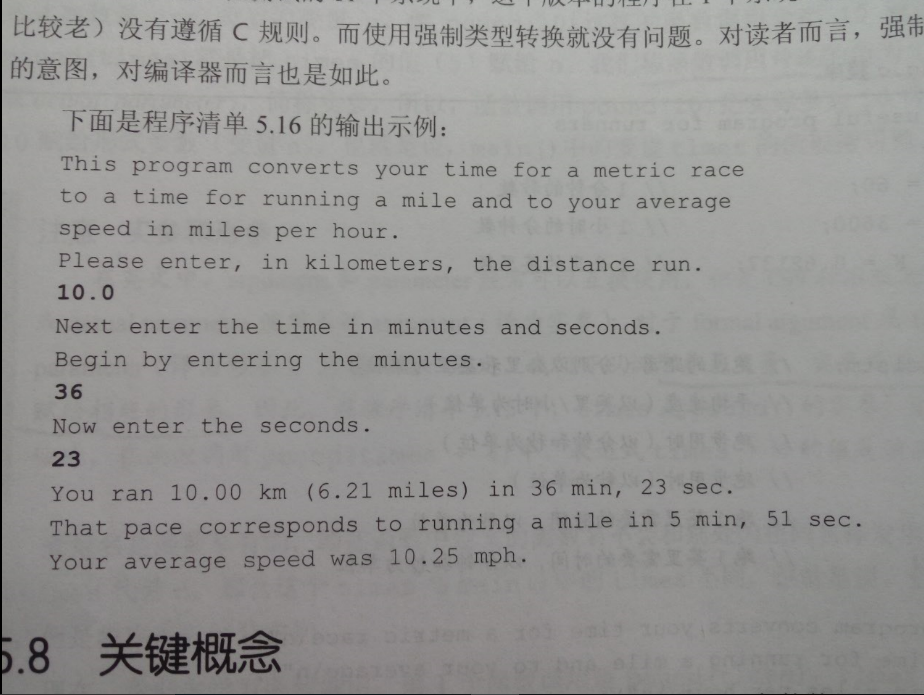2016-11-03 04:18

# c primer plus 第六版第5章程序清单5.16的问题

#include
const int S_PER_M = 60;
const int S_PER_H = 3600;
const double M_PER_K = 62137;
int main(void)
{
double distk, distm;
double rate;
int min, sec;
int time;
double mtime;
int mmin, msec;

``````printf("this program converts your time for a metric race\n");
printf("to a time for running a mile and to your average\n");
printf("speed in miles per hour.\n");
scanf("%lf", &distk);
printf("next enter the time in minutes and seconds.\n");
printf("begin by entering the minutes.\n");
scanf("%d", &min);
printf("now enter the seconds.\n");
scanf("%d", &sec);

time = S_PER_M*min + sec;
distm = M_PER_K*distk;
rate = distm / time*S_PER_H;
mtime = (double)time / distm;
mmin = (int)mtime / S_PER_M;
msec = (int)mtime % S_PER_M;

printf("you ran %1.2f km (%1.2f miles) in %d min,%d sec.\n",
distk, distm, min, sec);
printf("that pace corresponds to running a mile in %d min,",
mmin);
printf("%d sec.\nyour average speed was %1.2f mph.\n", msec,
rate);
getchar();
getchar();
return 0;
``````

}

this program converts your time for a metric race
to a time for running a mile and to your average
speed in miles per hour.
10.0
next enter the time in minutes and seconds.
begin by entering the minutes.
36
now enter the seconds.
23
you ran 10.00 km (621370.00 miles) in 36 min,23 sec.
that pace corresponds to running a mile in 0 min,0 sec.
your average speed was 1024705.45 mph.distm,min,sec,mmin,msec,rate返回的数据都不对，程序检查了很多遍。

• 点赞
• 写回答
• 关注问题
• 收藏
• 邀请回答